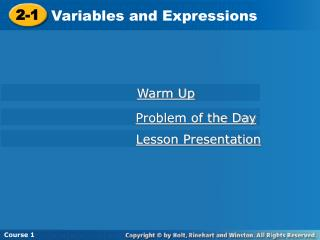DownloadDownload PresentationCourse 1

# Course 1

Télécharger la présentation## Course 1

- - - - - - - - - - - - - - - - - - - - - - - - - - - E N D - - - - - - - - - - - - - - - - - - - - - - - - - - -
##### Presentation Transcript

1. Course 1 2-1 Variables and Expressions Warm Up Problem of the Day Lesson Presentation Course 1

2. Warm Up Simplify. 1. 4 + 7  3  1 2. 87  15  5 3. 6(9 + 2) + 7 4. 35  7  5 24 84 73 25

3. 4 31 52 Problem of the Day How can the digits 1 through 5 be arranged in the boxes to make the greatest product? 

4. Learn to identify and evaluate expressions.

5. Vocabulary variable constant algebraic expression

6. A variable is a letter or symbol that represents a quantity that can change. A constant is a quantity that does not change.

7. Algebraic Expressions NOT Algebraic Expressions An algebraic expression contains one or more variables and may contain operation symbols. So p 7 is an algebraic expression. 150 + y 85 ÷ 5 35 w + z 10 + 3 5 To evaluate an algebraic expression, substitute a number for the variable and then find the value.

8. y 5  y 16 80 27 y = 27; 5  = y = 35; 5  = 35 Additional Example 1A: Evaluating Algebraic Expressions Evaluate the expression to find the missing values in the table. Substitute for y in 5  y. y = 16; 5  16 = 80 27 135 135 175 35 175 The missing values are 135 and 175.

9. z z  5 + 42 Substitute for z in z  5 + 42. 20 20 45 60 Additional Example 1B: Evaluating Algebraic Expressions Evaluate the expression to find the missing values in the table. z = 20; 20  5 + 16 = 20 25 z = 45; __  5 + 16 = __ 45 25 60 28 z = 60; __  5 + 16 = __ 28 The missing values are 25 and 28.

10. x x  9 18 2 x = 36;  9 = 36 x = 54;  9 = 54 Check It Out: Example 1A Evaluate the expression to find the missing values in the table. Substitute for x inx  9. x = 18; 18  9 = 2 4 36 4 6 54 6 The missing values are 4 and 6.

11. z 8 z + 23 Substitute for z in 8  z + 23. 7 64 9 11 Check It Out: Example 1B Evaluate the expression to find the missing values in the table. z = 7; 8  7 + 8 = 64 9 80 z = 9; 8  __+ 8 = __ 80 11 96 z = 11; 8  __ + 8 = __ 96 The missing values are 80 and 96.

12. Instead of . . . You can write . . . 35 y Writing Math When you are multiplying a number times a variable, the number is written first. Write “3x” not “x3.” Read 3x as “three x.” You can write multiplication and division expressions without using the symbols  and . x 3 x 3 x(3) 3x 35 ÷ y

13. Additional Example 2: Evaluating Expressions with Two Variables A rectangle is 4 units wide. How many square units does the rectangle cover if it is 3, 4, 5, or 6 units long? Make a table to help you find the number of square units for each length. 3 x 4 = square units 12 16 4 x 4 = square units 16 20 5 x 4 = square units 20 24 6 x 4 = square units 24 The rectangle will cover 12, 16, 20, or 24 square units.

14. Check It Out: Example 2 A rectangle is 3 units wide. How many square units does the rectangle cover if it is 2, 3, 4, or 5 units long? Make a table to help you find the number of square units for each length. 2 x 3 = square units 6 9 3 x 3 = square units 9 12 4 x 3 = square units 12 15 5 x 3 = square units 15 The rectangle will cover 6, 9, 12, or 15 square units.

15. Lesson Quiz 1. Evaluate the expression to find the missing values in the table. 95 44 20 2. A rectangle is 6 units wide. How many square units does the rectangle cover if it is 2, 3, 4, or 5 units long? 12 18 24 30